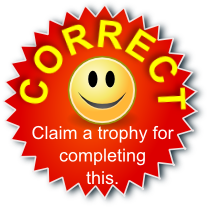# Parallel Graphs

Drag five yellow cards into each of the four rectangles.
All of the equations you place in each rectangle should be parallel to each other.

$$y=x$$

$$y=2x$$

$$y=2-x$$

$$y=5-3x$$

$$y=x+2$$

$$y=2x+5$$

$$y+x=7$$

$$y+3x=8$$

$$y=x-3$$

$$2x-7=y$$

$$y=-x+10$$

$$2y+6x=1$$

$$2y=2x-5$$

$$x= \frac12y$$

$$16=x+y$$

$$x = \frac{2-y}{3}$$

$$3x-3y=1$$

$$3y-6x=17$$

$$y= \frac13 - x$$

$$y=3(7-x)$$## Transum.org

This web site contains over a thousand free mathematical activities for teachers and pupils. Click here to go to the main page which links to all of the resources available.## More Activities:

Mathematicians are not the people who find Maths easy; they are the people who enjoy how mystifying, puzzling and hard it is. Are you a mathematician?

Comment recorded on the 9 October 'Starter of the Day' page by Mr Jones, Wales:

"I think that having a starter of the day helps improve maths in general. My pupils say they love them!!!"

Comment recorded on the 3 October 'Starter of the Day' page by S Mirza, Park High School, Colne:

"Very good starters, help pupils settle very well in maths classroom."

#### Three WaysCan you get to the target number by multiplying together four different one digit numbers? Can you do it in three different ways? There are nine levels to this online challenge and a virtual Transum Trophy available for each level.

## Numeracy

"Numeracy is a proficiency which is developed mainly in Mathematics but also in other subjects. It is more than an ability to do basic arithmetic. It involves developing confidence and competence with numbers and measures. It requires understanding of the number system, a repertoire of mathematical techniques, and an inclination and ability to solve quantitative or spatial problems in a range of contexts. Numeracy also demands understanding of the ways in which data are gathered by counting and measuring, and presented in graphs, diagrams, charts and tables."

Secondary National Strategy, Mathematics at key stage 3

## Go Maths

Learning and understanding Mathematics, at every level, requires learner engagement. Mathematics is not a spectator sport. Sometimes traditional teaching fails to actively involve students. One way to address the problem is through the use of interactive activities and this web site provides many of those. The Go Maths main page links to more activities designed for students in upper Secondary/High school.

## Teachers

If you found this activity useful don't forget to record it in your scheme of work or learning management system. The short URL, ready to be copied and pasted, is as follows:

Do you have any comments? It is always useful to receive feedback and helps make this free resource even more useful for those learning Mathematics anywhere in the world. Click here to enter your comments.For All: